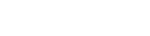# GDScript Looping

Looping in GDScript is done with either a for loop or a while loop.

## For Loop

The for loop sets an index value from a range of numbers or by iterating over an object such as an array or dictionary.

The range function let’s define a range of integers. The start number defaults to 0. The limit number is one more than the maximum count because that is traditionally how it is done since index values tend to start from zero rather than one.

We may also specify a positive or negative step value, the default is 1.

The statement body of the for loop is indented.

You can terminate the loop at any time with a break statement. This might be used to break out of a loop that is used to search for an item within a collection or text document.

To skip to the end of the statement block, we can use a continue statement.

Here are code examples showing the various ways to implement a for loop:

``````# loop for n = 0 to 7
for n in 8:
print(n)

# Using range
for n in range(8):
print(n)

# loop for n = 10 to 12
for n in range(10,13):
print(n)

# count down from 10 to 1
for n in range(10,0,-1):
print(n)

# loop for n = 2,4,6,8 in steps of 2
for n in range(2,9,2):
print(n)

# Iterate over string (array of characters)
for ch in "Hello":
print(ch)

# Iterate over an array of numbers
for x in [3,6,8,9]:
print(x)

# Iterate over items of a dictionary
var dict = { "x": 1, "y": 2, "z": 3 }
for key in dict:
# Insert the key and value into a text string
print("index: %s, value: %d" % [key, dict[key]])

# Using continue and break statements
for n in 9:
# Skip numbers below 3
if n < 3:
continue
# Break out of the loop for numbers above 5
if n > 5:
break
print(n)

``````

## While Loop

The while loop evaluates a boolean expression to decide whether to keep looping or exit the loop. Again, we may break out of the loop with a break statement or skip to the end of the statement block with a continue statement.

Here is some example code:

``````var fuel = 1000
var speed = 0

while fuel > 0:
speed += 0.12
fuel -= 1

print("Top speed = ", speed)

``````

Next we will look at GDScript Arrays.